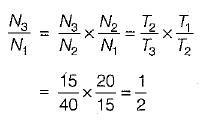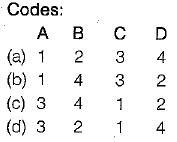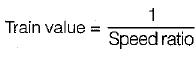Courses

# Test: Gear Trains - 1

## 10 Questions MCQ Test Topicwise Question Bank for Mechanical Engineering | Test: Gear Trains - 1

Description
This mock test of Test: Gear Trains - 1 for Mechanical Engineering helps you for every Mechanical Engineering entrance exam. This contains 10 Multiple Choice Questions for Mechanical Engineering Test: Gear Trains - 1 (mcq) to study with solutions a complete question bank. The solved questions answers in this Test: Gear Trains - 1 quiz give you a good mix of easy questions and tough questions. Mechanical Engineering students definitely take this Test: Gear Trains - 1 exercise for a better result in the exam. You can find other Test: Gear Trains - 1 extra questions, long questions & short questions for Mechanical Engineering on EduRev as well by searching above.
QUESTION: 1

### Consider a simple gear train having odd number of idler gears. The direction of motion of the driven gear will

Solution:

In simple gear train having odd number of ilder gears, the direction of motion of the driven gear will be same as that of the driving gear and vice- versa.

QUESTION: 2

### Consider a simple gear train consisting of three gear wheels with 20, 15 and 40 teeth respectively. The velocity ratio of third wheel to first wheel would be

Solution:QUESTION: 3

### If in a gear trains, the first wheel is a driver and the last wheel is a driven then train value is defined as the

Solution:

The reciprocal of the speed ratio (or velocity ratio) is known as train value of gear train.

QUESTION: 4

A gear train, in which atleast one of the gear axes is in motion relative to the frame, is known as

Solution:
QUESTION: 5

Match List-I with List-ll and select the correct answer using the code given below the lists:
List-I
A. Compound gear train
B. Epicyciic spur gear train
C. Worm and worm wheel gear train
D. Epicyciic bevel gear train

List- II
1. Automobile gear box
2. Automatic transmission of automobile
3. Speed reducer for lifts
4. Automobile differentialsSolution:
QUESTION: 6

The gear train usually employed in clocks is a

Solution:
QUESTION: 7

In a simple gear train, if the number of idle gears is odd, then the motion of driven gear will

Solution:

The speed ratio and the train value, in a simple train of gears, is independent of the size and number of intermediate gears. These intermediate gears are called idle gears, as they do not affect the speed ratio or train value of the system.

QUESTION: 8

The gear train usually employed in clocks is

Solution:

In reverted gear train, first and last gear is on the same axis such an arrangement has application on speed reducers, clocks and machine tools.

QUESTION: 9

A reverted gear train is one in which the output shaft and input shaft

Solution:

If the axes of the first and the last wheel of a compound gear coincide, it is called a reverted gear train.

QUESTION: 10

Train value of a gear train is

Solution: